Select Page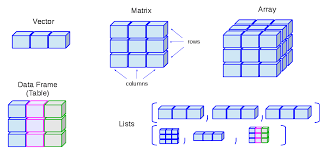Let us note right off the bat that this magical cube of 42, comprised of 27 Hebrew Letters, is in actuality a 3-d matrix, which in turn is called an array.  The 27 letters, or force archetypes, are no longer limited to a 2-dimensional line or even a 3 by 9 matrix.  This opens up their ability to interact on multiple dimensions at once, and our ability to analyze them. Remember, we are not folding them up into a cube. We are observing a 3-d projection of them from a higher dimensional hypercube that our minds cannot comprehend, much the way the 3-d cube was unfolded into a linear alphabet for our forefathers.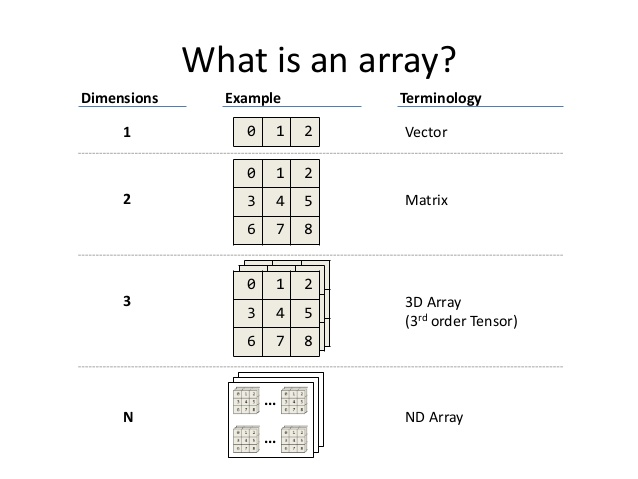Given that, we have a few further considerations on the magic cube of 42, or Merkabah.

After the 5th Element was Created, the 4 wings of the Alef(א) at the level of the Binah hyperspace dimension became the other 4 Elements of Creation, which were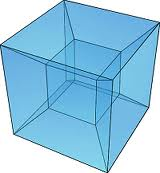presented to us as the first verse of the Shema or 15 Triplets with the concealed Upper 42-Letter Name; the 42-Letter Name or 14 Triplets; and the first 11 Triplets of Bereshit; and the 72 Triplets, collectively known as the 112 Triplets. Next came the Torah, whose 7 words and 28 letters hinted at the structure of the cube  within the 4 other Elements; and whose first 8 words or 33 letters that totaled 3003 in their numerical value hinted at the 33 structure of the cube itself; and whose 58 words, letters, and verses and whose 210 word values reflected the hypercube origin of the magical cube.

### Vectors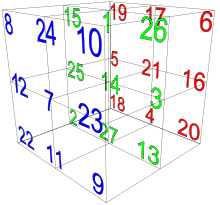Including the additional corner vector that pierces the central #14 cube, there are a total of 7 vectors emanating from each of the 8 corners. What is this like? The 7 dimensions (sefirot) emanating from the 8th dimension (Binah). There is a total of 14 vectors pivoting on the central #14 cube, reflecting the 14 chambers discussed in the previous section.  Altogether, there are a total of (56 + 14) = 70 vectors, fully reflective of the level of Zeir Anpin and Malchut inclusive. The 70 vectors plus the whole cube of 42 is (70 + 42) = 112, as in the 112 Triplets and the Unification of Elohim and YHVH (86 + 26), or alternatively Adonai, YHVH, and Ehyeh (65 + 26 + 21).

What is this like? It is like the 42nd Name (Triplet) of the 72 Names (triplets) whose numerical value is 70 and whose complete value is (70 + 42) = 112.

By the way, as Moshe pointed out to me, the 112th prime number is 613, as in the 613 mitzvot (precepts) detailed in the Torah. It is all connected.

How many Names are there in the 72 Names (Triplets)? With the two repeated ones, there are only 70.  Only 70! Or are there necessarily 70 in order to match the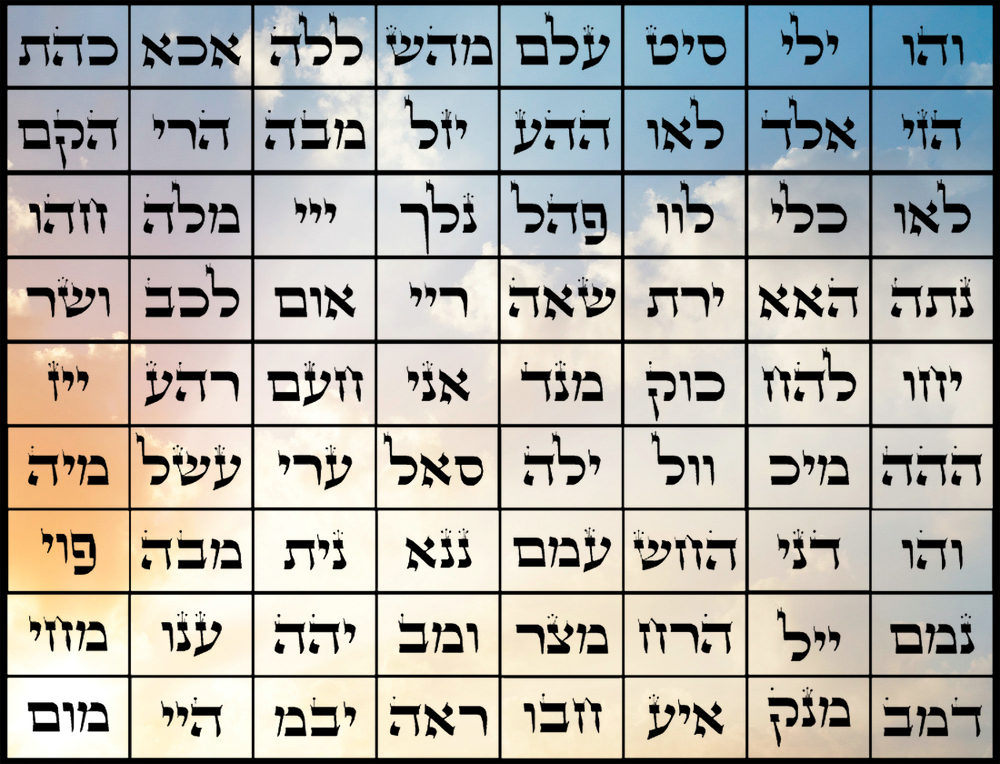70 vectors and 70 dimensions of unification. The Zohar took note of this and further explained that this is why there were 70 members of the Sanhedrin, 70 faces of the Torah, and 70 Nations and 70 languages of our world.

Regarding the first verse of the Shema that conceals the Upper 42-Letter Name, we can further understand that (15 Triplets + 27 letters in the Cube) = 42, and that (28 + 42) = 70, representing the three other Essential Elements (Forces) of the 5 of Creation. Moreover, (5 x 14) = 70.  Most of the vectors come in triplets themselves given the nature of a 33 cube. In other words, 3 parallel vectors or 9 letters in each plane. And what is the measure of each triplet vector? 126 or (3 x 42). And what is 126 but (112 + 14). Therefore, each of the triplet vectors is a conjunction of the 112 Triplets with the central core emanation of 14.

Similarly, the total numerical value of the 6 faces, 12 edges, 8 vertices, and 1 grand cube is 1120, (112 x 10). It is also (26.666 x 42). These (6 + 12 + 8 + 1) elements = (26 + 1) or 27 cube defining spiritual elements are missing only the concealed central cube of 14 that completes it and elevates it to Binah.

The letter Nun (נ) associated with the cube of 14, has a value of 50, as in the 50 Gates of Binah and the 50 years of Jubilee, representing complete completion. The Hebrew word for all (כל) has the numerical value of 50. The Torah instructed us in 2448 HC, the year we first received it, to count the Jubilee years, one for every 50 calendar ones. When we add the 14 to 1120, we get 1134, which as it turns out equals (27 x 42), the power of 42 infused in every letter of the Alef-bet. Thus, completed with the central power cube, there are now (27 + 1) = 28 defining cubic elements of the magic cube of 42, the 5th Essential Element of Creation.

Since the 27 letters of the cube total 378, the relationship between the whole cube and the central cube is 378/14 = 27.  The central cube is indeed 1/27th of the whole.

I must give special thanks to Rav Yehuda Tzvi Brandwein of blessed memory for his insights into these matters.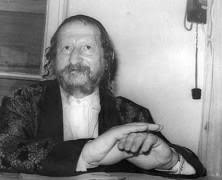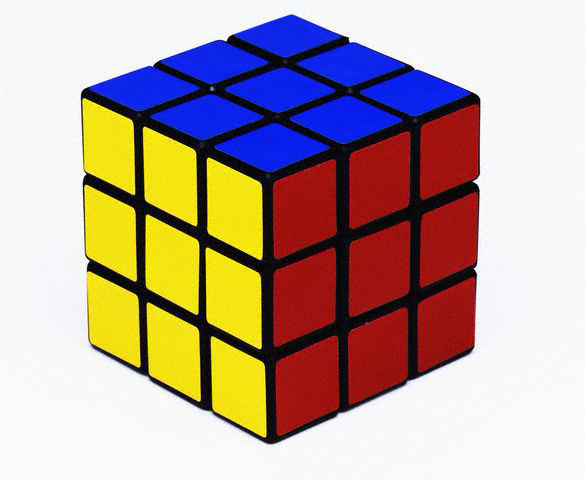The complete (ordinal and standard) numerical value of the 27 structural cubic elements is 20420, yet an odd thing occurs if we consider all 28 elements and discount the ordinal value (504) for the 12 edges.  I do not know why we would do that, but the total then becomes 19980, or 4 times the value of the 27 letters, 4995. It is also (6660 x 3) or (3330 x 6), reflective of the 666 Jubilee years from the giving of the Torah at Mt Sinai on 2448 HC and 5778 HC, when the cosmic window opened for the 3rd time.

The net difference between the two equations above is 440, and 440 + One(1) = 441, Emet, Truth.

The ordinal value (504) for the 12 edges matches the ordinal value (504) for the 12 straight diagonals. Please note that there are also 4 cross cube diagonals. This is by design since 504 is (12 x 42).  What is also by design is that the 33rd unique or 47th actual doubled word in the Torah has a numerical value of 504 each, and there are 50 of them in total 25 to the midpoint location of the doubled word and 25 after it. By the way, the 42nd doubled word is the YHVH (יהוה יהוה). It is also the 30th unique double word in the Torah. And what is this like? It is like the 30 times the name Yehuda of numerical value 42 appears in the Torah, and it is like the letter Lamed (ל) of numerical value 30 and of ordinal value 12 for a complete value of 42.

Understand which came first.

And what is this like?

# The Sword of Moses

It is like the Song of the Sea that we recite on the 7th Night of Pesach and should recite together every Shabbat. It is written in 30 rows and has the sword of Moses, the verse of 42 letters, right smack in the middle at Exodus 15:11. It was 42 verses after the start of chapter 14, where G-d told Moses to tell the Israelites to camp at Freedom Valley, Pi (פי) HaChirot(החירת) beside the Red Sea. Halfway to the verse, 21 verses later, He split that Sea.    Pi (פי) HaChirot(החירת) breaks down numerically to 5’708 as in 5708 HC, the year Israel became a nation. It is of note that chapter 14 and Exodus 15;11 together reflect 3 of the Essential Elements: the 14 Triplets of the 42-Letter Name; the 15 Triplets of the Shema’s first verse and the 11 Triplets of Bereshit, all the while encapsulating the 4th Element,  72 Triplets revealed at the splitting of the Sea.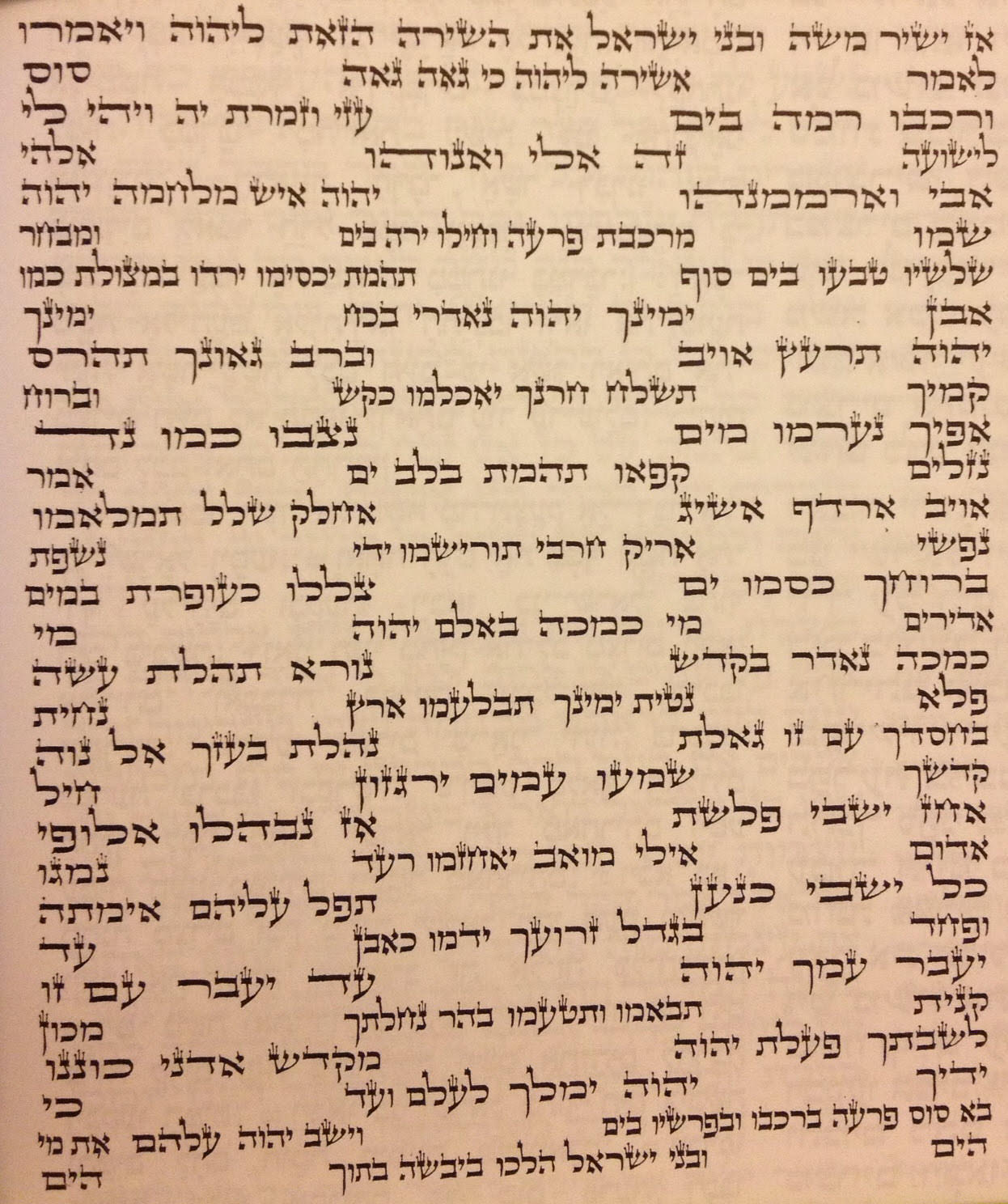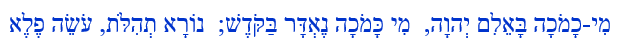These are deep revelations.  Taken in reverse, the sword sharpens to a point, as pheleh (פֶלֶא) backwards is Aleph (אלֶפֶ), the first letter of the Hebrew Alphabet (א), expressing the four sides of the sword spreading out from the central point, like a 4-sided pyramid seen from the top.  The handle of this sword is the 42nd triplet (מִיכָ) of the 72 Triplets used to split the Red Sea.   When the time comes, this 42-letter sequence will be reversed and the Mem(מ) in (מִיכָ) will become a final Mem sofit (ם), representing 4 vavs (ו) amongst other things.  We mention the 4 Vavs (ו ו ו ו) because when taken in reverse, the numerical value of this 42-letter verse when the kolel of 42 is removed is 6666, representing the 4 vavs, each of numerical value 6.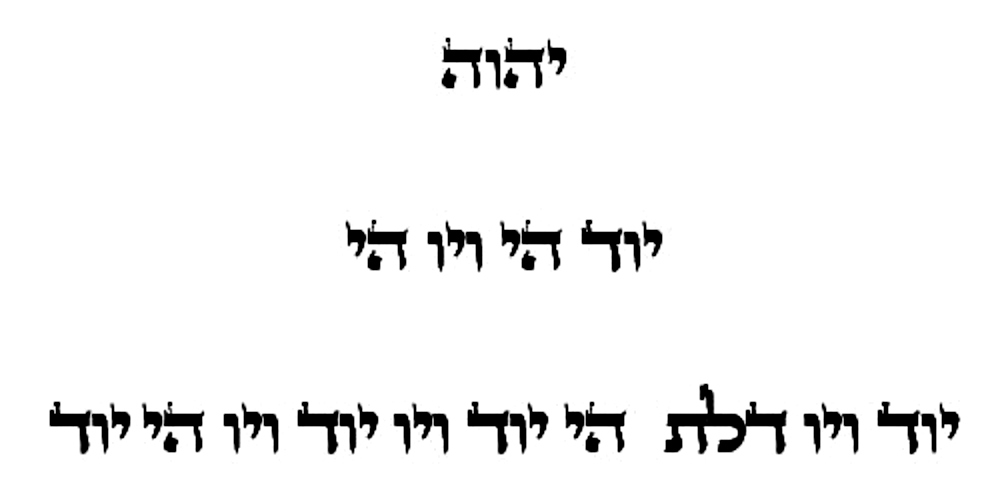As we just discussed, the Upper 42-Letter Name of G-d has the numerical value of 708, while the Sword of Moses without removing the kolel has a numerical value of 6708, so it is doubtful this is coincidental, nor is it coincidental that there are 670 paragraphs in the Torah or that 67 is the value of Binah, the upper level sefira (dimension) to which Moses connected.

### The Hidden Gateway and the Chariot

Now notice that the 42-letter verse of the sword in reverse is split into 28 letters and 14 letters. This mirrors the 42-Letter Name of G-d integrated in the first 42 Letters of Bereshit in that there are 28 letters in Bereshit’s first verse and 14 letters that follow to complete the Name.Before even Adam, before the beginning of time, the 42-Letter Name was converted into the first 42 letters of the Torah.  For simplicity’s sake, we can envision that conversion as 42 wheels on a combination lock, each engraved with 22 Hebrew letters, and when we turn those 42 dials to just the right combination, all the gates open. Except that 3 of those dials are never turned, because 3 letters never changed, the 8th, 18th and 40th.  This is a code unto itself.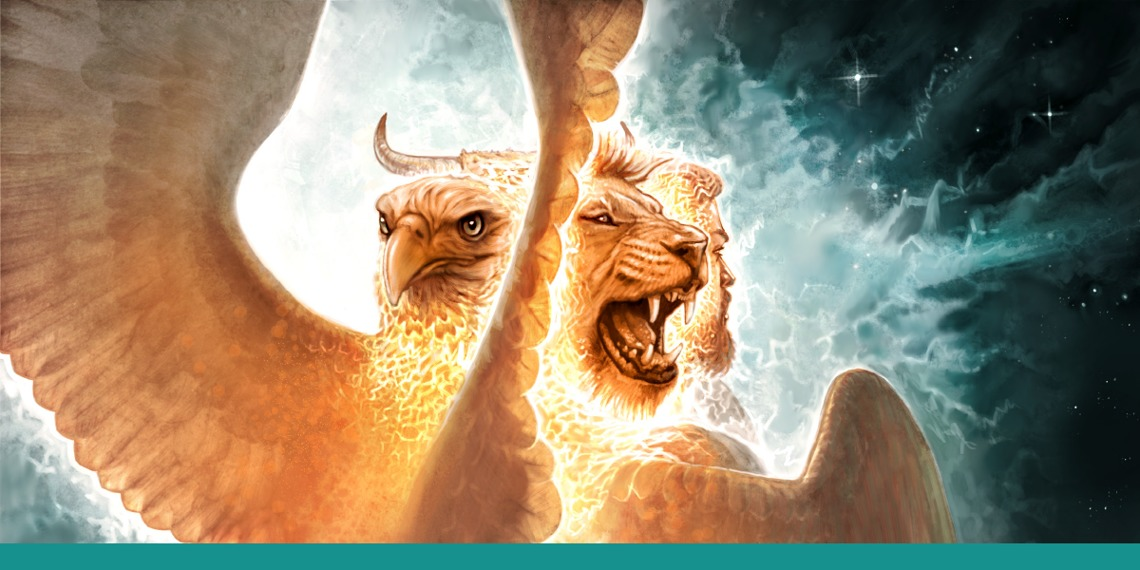Furthermore, those 3 letters are the Shin(ש), Resh(ר) and Vav(ו), which form the 32nd of the 72 Names(ושר).  Permutations of these 3 letters form the Hebrew word for Ox(שור), a deep kabbalistic principle that we will not get into now, but it is one of the 4 faces of the Merkavah.   We find all 3 of those letters (#21, #20, and #6) connected to each other on the same (rear) face of the magic cube of 42. Collectively the 3 letters have the numerical value of 506, as in the complete value of Moshiach Ben David, the first line of the 42-Letter matrix, the kabbalistic term faces (פרצופים), and the sum of the initials in the 9 spiritual sefirot.

The other oddity in the conversion of the 42-Letter Name into the first 42 letters of the Torah is the skipping of a specific letter Hei (ה), the second Hei (ה) of (היתה), the 37th letter of the Torah, of numerical value 5.  This is the gateway.  It is also found on the same face as the Ox (שור), spelling out “The Ox (השור),” and the sum of their Torah ordinal values is 52.

Understand which came first.

### Life and the Tree-of-Life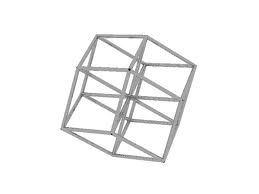At the center of the magic cube of 42 is the central cube of 14 and letter Nun ) of 50 for a complete value of 64, which can be said to coincide with the 64 codon (Triplets) in our DNA coding and also the 64 codon (Triplets) in our RNA coding, our physical essence and our source of physical life.  In the Zohar, Vayikra 27:240 it refers to the Torah verse Bereshit 2:09: “ ‘The Tree of Life in the midst of the garden,’ called life.” The garden (הגן) has a numerical value of 708, which is purposely the same as the Upper 42-Letter Name of G-d. What can we see at the center in the midst of the garden(708)? The cube of life, the Tree-of-life.

The magic cube of 42, refers to the Upper 42-Letter Name of G-d, through which as explained the Arizal, all our prayer gets processed. It is the garden, the 5th Element of Creation. What other schematic do we find continuing in the subsequent verse Bereshit 2:10? “A river flowed out of Eden to water the garden. From there it divided and became four major rivers.” Binah flowed from the garden and became 4 (Essential Elements /Forces of Creation).

Where else to we find the garden and the 5th Element of Creation, the Upper 42-Letter Name of G-d, as 708? We find it in the date Israel became a nation, the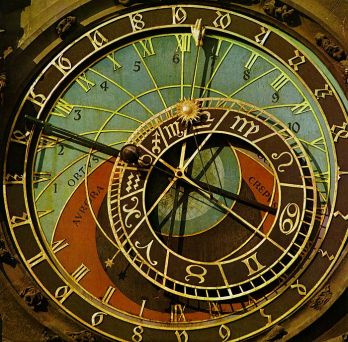year 5708 HC. Why was it prophesied to be 70 years before 5778 HC? Because the of 70 sefirot in the full unified Zeir Anpin/Malchut, the same 70 dimensions represented by the 70 vectors in the magic cube of the Upper 42-Letter Name of G-d.

What was the year 5778 HC in the Western Calendar? 1948 CE, and the cube root of 48 is 3.63424…, H’Moshiach (363) and Moshiach Ben David (424).   If you recall, in our previous section in discussing the connection between the 33 magic cube of 42 and the 72 Triplets, we said that the sum of the ordinal values all the facial rows, columns, and diagonals is (756 + 756 + 504) = 2016.  Well, 2016 represents (42 x 48).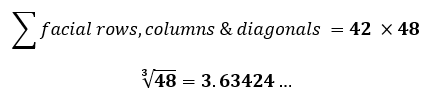### U’Malchut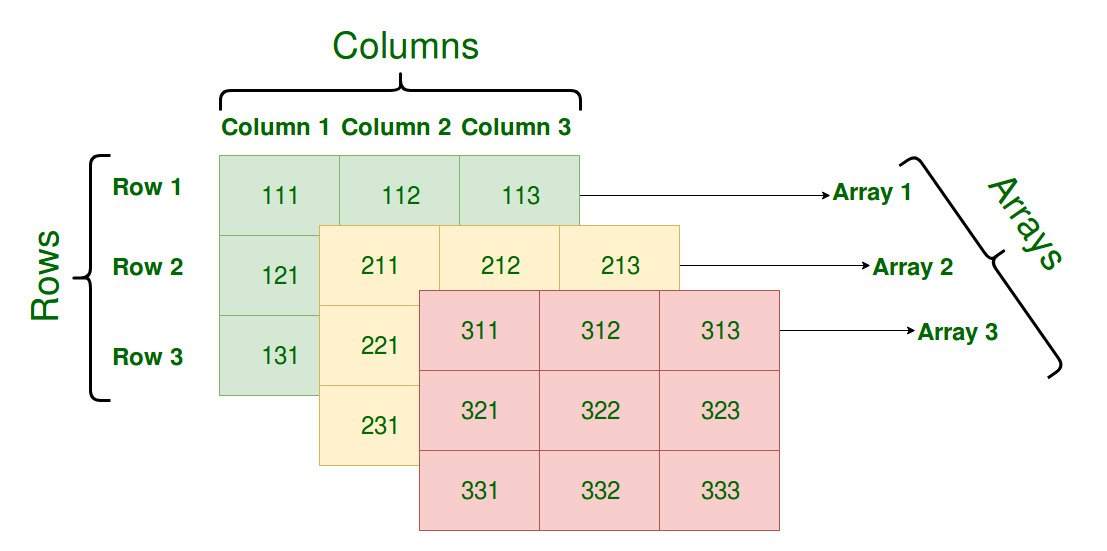We have examined the interior and faces (partzufim) of the magic cube of 42 separately. Now, as a whole cube of 27 numbered unit cubes, there are 9 columns, 18 rows, 16 diagonals and 1 grand cube for a total of 44, equal to the candles we light on Chanukah, when we connect with the light by drawing down the river of Binah. The total value of those 44 elements is 2184 or (42 x 52).  The value 52 is the numerical value of the aspect of the Tetragrammaton associated with Malchut (יוד־הה־וו־הה). Moreover, The average of those 44 spiritual channels is (2184/44) = 49.6363, which could be representative of 496 (Malchut) and 363 (H’Moshiach).

There is another way to look at the vector system of the cube and that each vector can start at either end of the given Triplet, meaning that the 9 columns, 18 rows, 16 diagonals become 18 columns, 36 rows, 32 diagonals for a total of 86, as in Elohim, and they have a total gematria of (42 x 86) = 3612.  And like the unification of (86 + 26) = 112, the unification of those 86 vectors (Triplets) with the Upper 42-Letter Name expansion of the YHVH, 708, gives us (3612 + 708) = 4320.

Is this an alignment with the 432,000 stones of 1 cubic cubit each for the outer border wall of the Future Holy Temple?

Is this an alignment with the operating frequency of Binah within our world? Is it 432 Hz as we have identified before?

On the front face of the magic cube of 42, we have the numbers 12 and 7 side by side. This might be an allusion to the 7 words of the first verse of the Torah and the 12 words in the last. This is the same face of the cube that can be split into 54 and 72. The Torah’s first word Bereshit (בראשית) when connected to the last word Israel (ישראל) completes the annual cycle of 54 portions, and this circle forms an 11-letter phrase, “Israel in the Beginning.”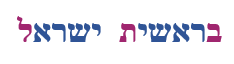Separating out the first, middle and last letters (בלת), of numerical value 432, we are left with two identical words, “(אשרי) Ashrei,” meaning blessing/happy. Within each of those words, and in the center of both Bereshit (בראשית) and Israel (ישראל) is the name/word Asher, as in “I am that I am” (אהיה־אשר־אהיה).  Thus, the ordinal value at the core (אשר) of both Israel, Bereshit, and “I am that I am,” is 42.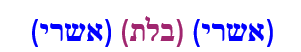Is the total 4320 of the 5th Essential Element in alignment with the design of the solar system, given that number 432 is reflected in the ratios of the Sun, Earth,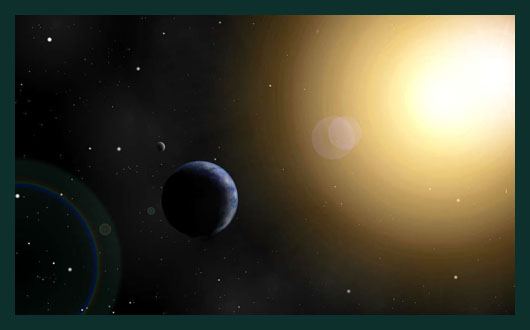and the moon? The diameter of the moon 2,160 miles is 5 times 432, or half of 4320.  The diameter of the sun is 864,000 miles (200 x 4320). Moreover, we can ask if the total 4320 of the 5th Essential Element in alignment with the 24 hours in an earth day, which is composed of 86,400 seconds or (20 x 4320).

Then with every blessing we say and utter the words Atah Adonai (אתה יהוה) we are invoking that special frequency, 432 Hz, since they have the combined gematria of (406 + 26) = 432.

All these revelations are just considerations of the numbers, the energetic impressions or reflections on our world. They represent the 27 Hebrew letters, which in turn represent the 27 forces or pressure modalities upon Binah and Zeir Anpin that Created and sustain our world. The numbers help us understand the necessary order and interconnections between the forces, yet it is through the letters themselves that we must ultimately connect. This is why we began this series with the Alef-bet.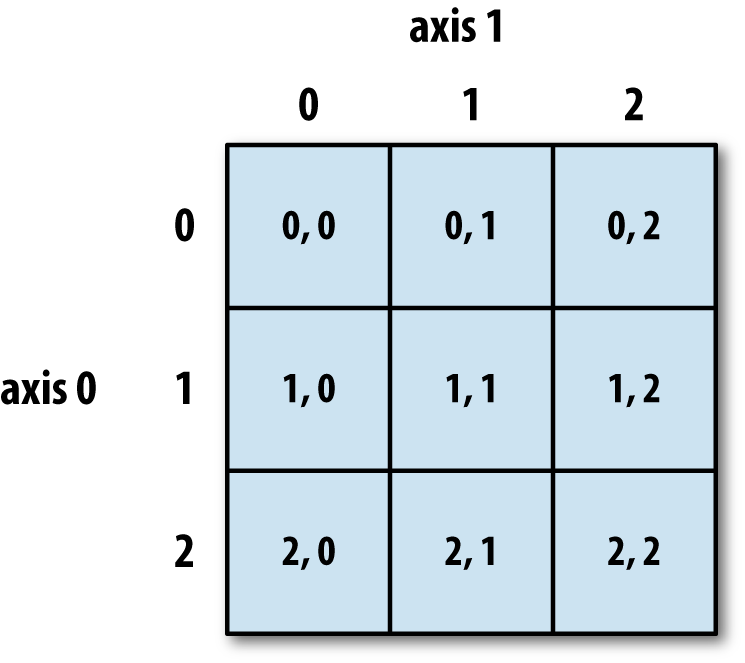The 27 letters of the Alef-bet can form a 3 x 9 matrix and give us 9 Triplets, but when wrapped tight in a 33 three-dimensional matrix of cube, we get 86 Triplets, a whole other level of complexity. There are two simple equations to keep in mind at the core of all this. The total ordinal value of the 27 letters is 378, giving us (9 x 42) = 378, meaning each letter is tethered to the 42-letter Name. And the second equation is (378/27) = 14, meaning that every letter is also tethered to the core cube of 14.  Then, let us not forget that 9/.123049… or 9/(42-Letter Name) = 73.1415777… = (70 + Pi). The value of Chochma, the 9th sefira (dimension) is 73.

The 7th day of Pesach is a time to connect to Moshiach. Together, we can unlock the power of this magic cube.

Chag Pesach Sameach

Ezra